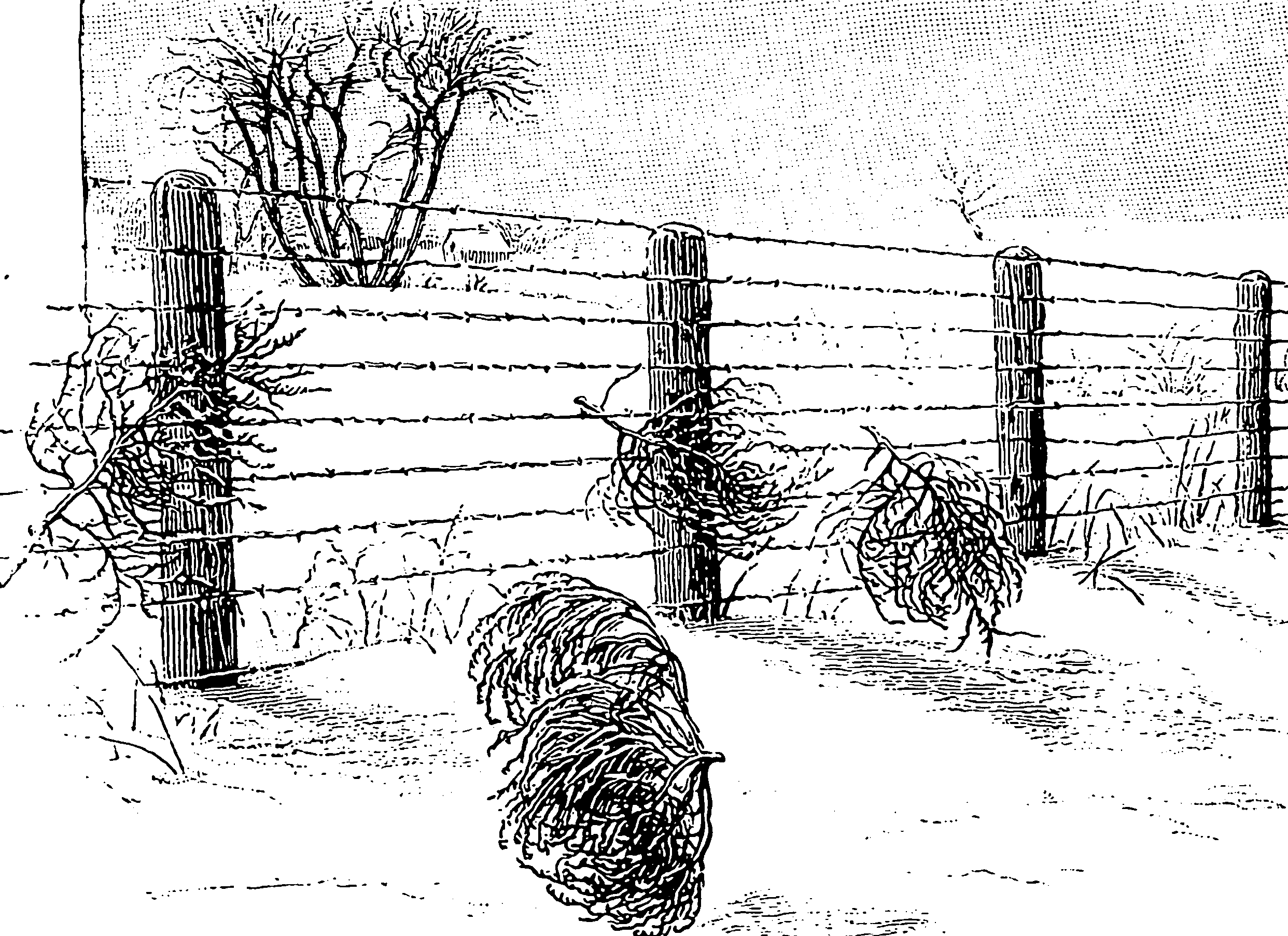Taking gradients through integrals using randomness. A thing with similar name but which is not the same is Stochastic Gradient MCMC which uses stochastic gradients to sample from a target posterior distribution. Some similar tools and concepts pop up in both uses.

## Score function estimator

A.k.a. REINFORCE, all-caps, for some reason. Could do with a decent intro. TBD.

A very generic method that works on lots of things, including discrete variables; however, notoriously high variance if done naïvely.

For unifying overviews see and the Storchastic docs.

### Rao-Blackwellization

Rao-Blackwellization seems like a natural trick gradient estimators. How would it work? Liu et al. (2019) is a contemporary example; I have a vague feeling that I saw something similar in Reuven Y. Rubinstein and Kroese (2016). TODO: follow up.

## Parametric

I can imagine that our observed rv $${\mathsf{x}}\in \mathbb{R}$$ is generated via lookups from its iCDF $$F(\cdot;\theta)$$ with parameter $$\theta$$: $\mathsf{x} = F^{-1}(\mathsf{u};\theta)$ where $$\mathsf{u}\sim\operatorname{Uniform}(0,1)$$. Each realization corresponds to a choice of $$u_i\sim \mathsf{u}$$ independently. How can I get the derivative of such a map?

Maybe I generated my original variable not by the icdf method but by simulating some variable $${\mathsf{z}}\sim F(\cdot; \theta).$$ In which case I may as well have generated those $$\mathsf{u}_i$$ by taking $$\mathsf{u}_i=F(\mathsf{z}_i;\theta)$$ for some $$\mathsf{z} \sim F(\cdot;\theta)$$ and I am conceptually generating my RV by fixing $$z_i\sim\mathsf{z}_i$$ and taking $$\phi := F^{-1}(F(z_i;\theta);\tau).$$ So to find the effect of my perturbation what I actually need is

\begin{aligned} \left.\frac{\partial}{\partial \tau} F^{-1}(F(z;\theta);\tau)\right|_{\tau=\theta}\\ \end{aligned}

Does this do what we want? Kinda. So suppose that the parameters in question are something boring, such as the location parameter of a location-scale distribution, i.e. $$F(\cdot;\theta)=F(\cdot-\theta;0).$$ Then $$F^{-1}(\cdot;\theta)=F^{-1}(\cdot;0)+\theta$$ and thus

\begin{aligned} \left.\frac{\partial}{\partial \tau} F^{-1}(F(z;\theta);\tau)\right|_{\tau=\theta} &=\left.\frac{\partial}{\partial \tau} F^{-1}(F(z-\theta;0);0)+\tau\right|_{\tau=\theta}\\ &=\left.\frac{\partial}{\partial \tau}\left(z-\theta+\tau\right)\right|_{\tau=\theta}\\ &=1\\ \end{aligned}

OK grand that came out simple enough.

TBC

## Tooling

van Krieken, Tomczak, and Teije (2021) supplies us with a large library of pytorch tools for stochastic gradient estimation purposes, under the rubric Storchastic. (Source.). See also Deepmind’s mc_gradients.

## Optimising Monte Carlo

Let us say I need to differentiate through a monte carlo algorithm to alter its parameters while holding the PRNG fixed. See Tuning MC.

### No comments yet. Why not leave one?

GitHub-flavored Markdown & a sane subset of HTML is supported.